# Paper Cutting - Non Verbal Reasoning questions

## Paper Cutting - Non Verbal Reasoning questions

Q1. Choose a figure which would most closely resemble the unfolded form of Figure (B).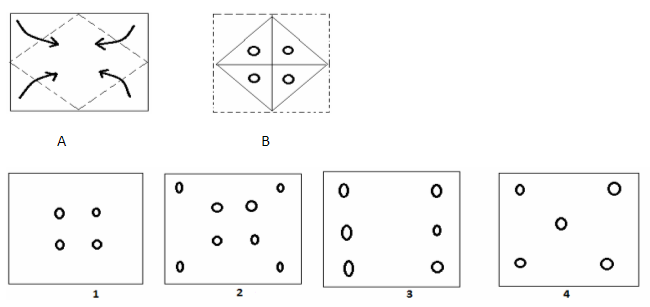a. 1
b. 2
c. 3
d. 4

Q2. Choose a figure which would most closely resemble the unfolded form of Figure (C).a.1
b.2
c.4
d.3

Q4. Choose a figure which would most closely resemble the unfolded form of Figure (C).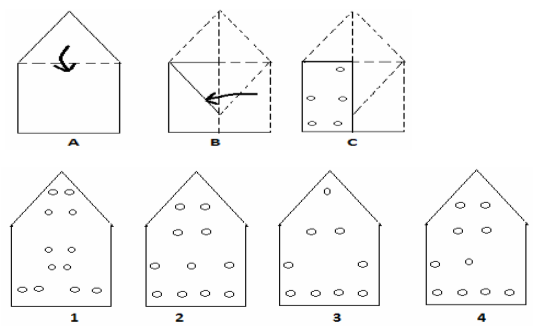a.2
b.3
c.1
d.4

Q5. Choose a figure which would most closely resemble the unfolded form of Figure (D).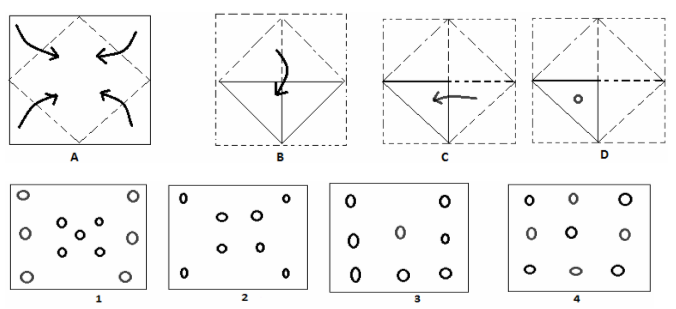a.1
b.3
c.2
d. None of these

Q6. Choose a figure which would most closely resemble the unfolded form of Figure (C).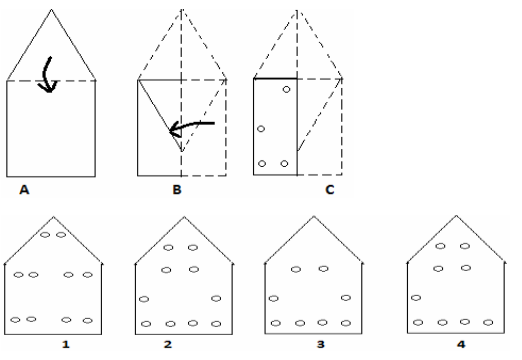a.3
b.4
c.2
d.1

Q7. Choose a figure which would most closely resemble the unfolded form of Figure (C).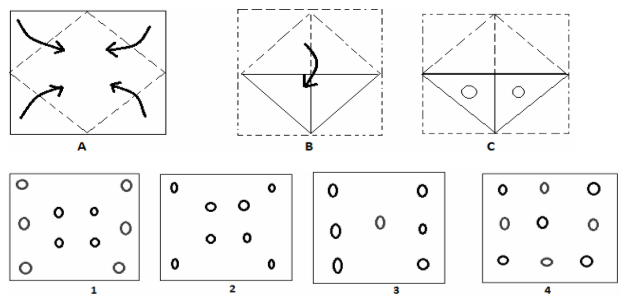a.1
b.2
c.3
d.4

Q8. Choose a figure which would most closely resemble the unfolded form of Figure (C).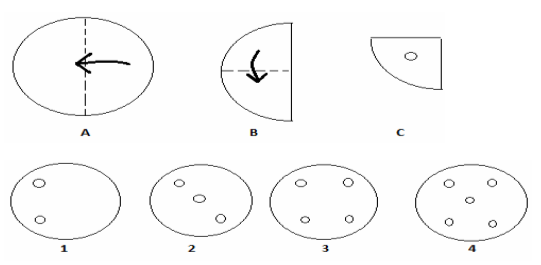a. 2
b. 1
c. 3
d. 4

Q9. Choose a figure which would most closely resemble the unfolded form of Figure (C).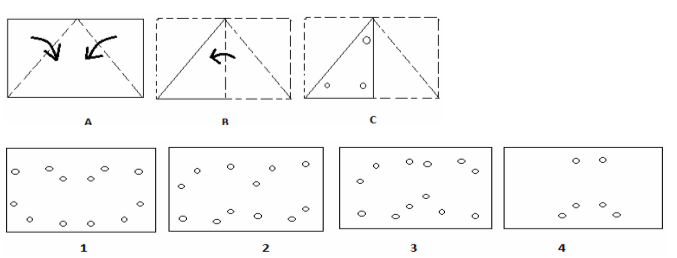i. 2
b. 3
c. 1
d. 4

Q10. Choose a figure which would most closely resemble the unfolded form of Figure (C).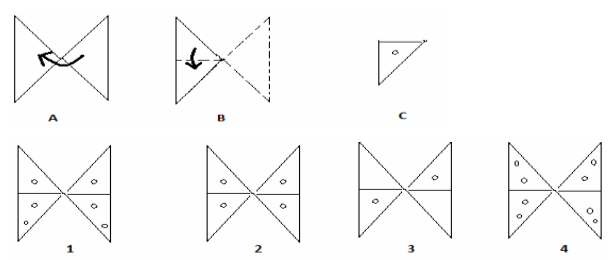a. 1
b. 2
c. 3
d. 4

Q11. Choose a figure which would most closely resemble the unfolded form of Figure (C).i. 1
ii. 2
iii. 4
iv. 3

Q12. Choose a figure which would most closely resemble the unfolded form of Figure (D).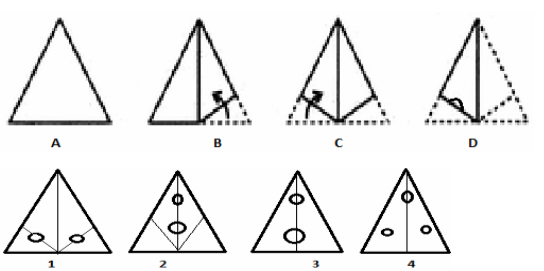a. 1
b. 2
c. 3
d. 4

Q13. Choose a figure which would most closely resemble the unfolded form of Figure (C).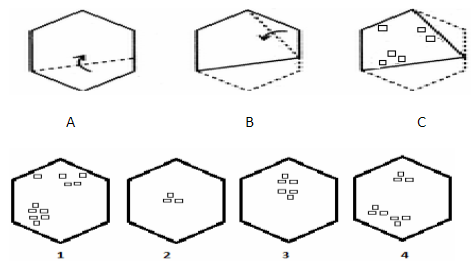i. 2
ii. 1
iii. 3
iv. 4

Q14. Choose a figure which would most closely resemble the unfolded form of Figure (C).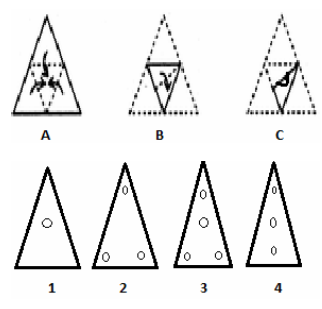i. 1
ii.2
iii. 3
iv. 4

Q15. Choose a figure which would most closely resemble the unfolded form of Figure (C).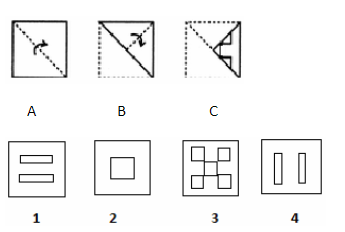i. 2
ii. 1
iii. 3
iv. 4

Q16. Choose a figure which would most closely resemble the unfolded form of Figure (C).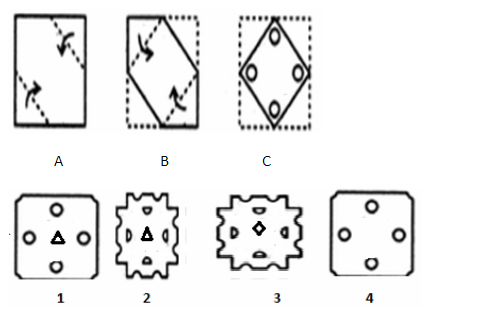i. 1
ii. 3
iii. 4
iv. None of these

Q17. Choose a figure which would most closely resemble the unfolded form of Figure (C).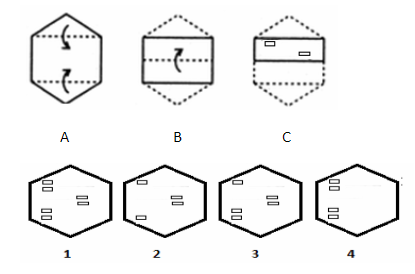i. 1
ii. 2
iii. 3
iv. 4

Q18. Choose a figure which would most closely resemble the unfolded form of Figure (C).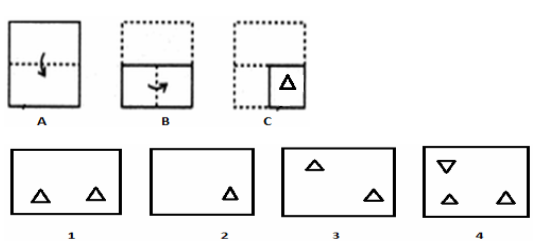i. 1
ii. 4
iii. 2
iv. None of these

Q19. Choose a figure which would most closely resemble the unfolded form of Figure (C).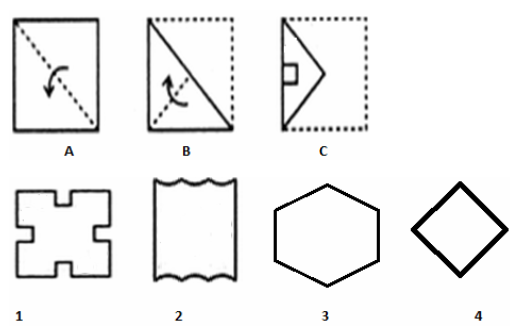i. 2
ii. 1
iii. 3
iv. None of these

Q20. Choose a figure which would most closely resemble the unfolded form of Figure (C).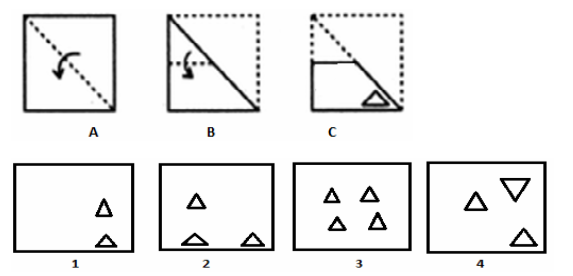i. 4
ii. 2
iii. 3
iv. None of these